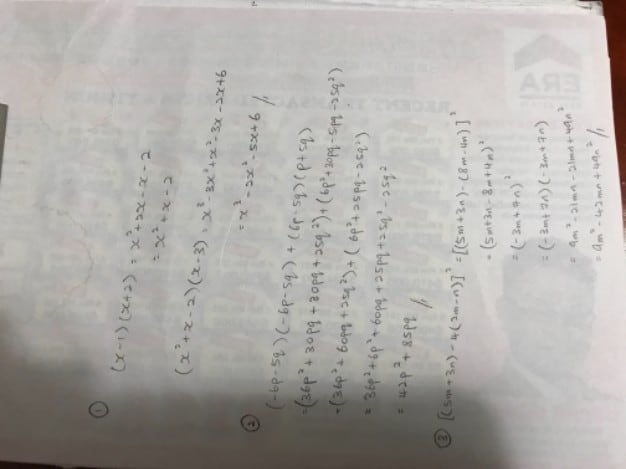Question

Hi, would really appreciate if I could get help with these algebra questions. Thanks!

Expand the following: (x-1)(x+2)(x-3)

Expand and simplify each of the following:

(-6p-5q)²+(6p-5q)(p+5q)

[(5m+3n)-4(2m-n)]²

Thanks again! 🙂